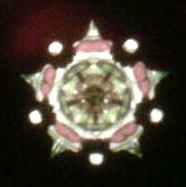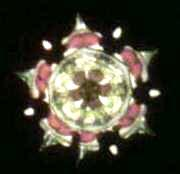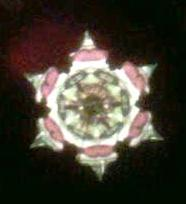## Kaleidoscope Mirror Systems - 2-Mirror Systems

2- Mirror Systems

The 2-miiror system is configured in a triangular shape. Two sides are mirrors and the third side blackened.

It produces one central image in the middle of the viewing field.  That central image is circular in shape and is a reflection of the objects being viewed.

The angle of the 2-mirrors determined the number of reflections that comprise the image circle.   The tighter the angle the higher the number of reflections (called folds).  Every two folds forms a point.  An image circul with 5 points will have 10 folds or reflections.

The following mathmatical formulars will calculate he number of folds and points:

• Folds = 360 didided by the angle of the 2 mirrors
• Points = 180 divided by the angle of the 2 mirrors

Here are the calculations for the common angles used with 2-mirror systems:

 Angle of Mirrors Folds Points 90 Degrees 4 2 60 Degrees 6 3 45 Degrees 8 4 36 Degrees 10 5 30 Degrees 12 6 25.7 Degrees 14 7 22.5 Degrees 16 8 20 Degrees 18 9 18 Degrees 20 10 16.3 Degrees 22 11 15 Degrees 24 12 12 Degrees 30 15 10 Degrees 36 18 9 Degrees 40 20 6 Degrees 60 30 5 Degrees 72 36

The image created by a 2-mirror system will be symmetrical if the mirror angle is an even divider of 360 degrees.  If the angle doesn't divide evenly into 360, for example 50 degrees (360 / 50 = 7.2), it will produce a non-symmetric pattern.

Below are three pictures of the same image inside the same scope.   The mirror angles are at 36 degrees (5 points), 33 degrees (5.5 points) and 30 degrees (6 points).  Notice the half point on the bottom of the center picture.